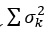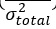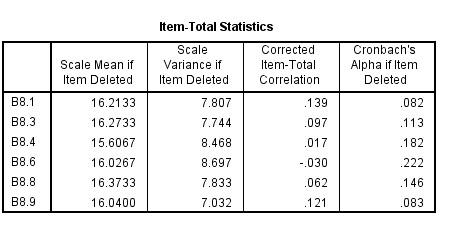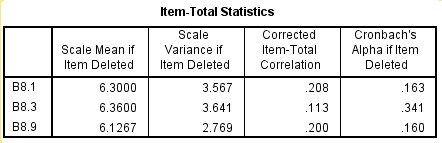# How to handle a negative Cronbach’s alpha value?

By Riya Jain & Priya Chetty on October 6, 2021

Primary data that is presented in the numeric form, such as survey responses, often requires reliability analysis. Especially if there are many independent variables for a dependent variable. For instance, in a study where the aim is to investigate how; job stress, job responsibilities, working hours, salary and, working environment impact job satisfaction. And the primary data is collected through a survey of employees, then a regression test must be applied. In such cases, chances of error in the survey data can increase. To remove the error and establish the reliability of the instrument (i.e. survey questionnaire), Cronbach’s alpha test is applied. The ideal Cronbach’s alpha value is at least 0.7 (Taber, 2018). However, sometimes Cronbach’s alpha value is negative, i.e. below 0. This article explains how to handle negative Cronbach’s alpha value so that it is turned acceptable.

## Introduction to Cronbach’s alpha test

Cronbach’s alpha value is computed by using the below-stated formula (Ritter, 2010).

Wherein:

• K= number of items,
•= k item score variance sum
•= scores variance on total measurement.

## Understanding negative Cronbach’s alpha value

The negative Cronbach’s alpha indicates the existence of negative reliability. It means that there is a negative average covariance among the items. This negative value is due to the derivation of data from the items representing different scales. True population value though is true but the existence of sampling error in the dataset could result in negative average covariance and hence the derivation of negative alpha value (Ursachi, Horodnic, & Zait, 2015). Hence, there are two reasons why Cronbach’s alpha value is negative:

1. sampling error
2. incompetence of the collected sample in measuring the respective questionnaire instrument.

## What to do when the Cronbach’s alpha value is negative?

There are two ways to treat data when the Cronbach’s alpha value is negative (Ursachi et al., 2015):

1. Eliminate the statements making the construct ineffective.
2. Collect the data from a new sample.

### Eliminate statements making construct ineffective for improving Cronbach’s alpha value

Cronbach’s alpha value is derived in two ways:

1. Item-wise: deriving value for every item in a scale or construct
2. Overall: the combined value of all items in a scale or construct

When Cronbach’s alpha value of an overall scale or construct is negative or suboptimal, the first step is to derive the item-wise value. By doing this, the researcher can eliminate the items for which the value is negative. This will improve the overall reliability or score.

### Collect a new dataset for improving the Cronbach’s alpha value

Sampling error, i.e. inefficient sample size or sample profile can also result in a negative Cronbach’s alpha value. This should be handled by completely changing the dataset. The researcher should eliminate the chances of bias and human error to a greater extent by ensuring that the sampled population are appropriate for the study. Hence, the new sample should have some relationship i.e. should capture the perception of respondents based on a single scale. This will help to improve the results with a negative Cronbach’s alpha value.

## Understanding it with a sample case

Topic: Sustainable practices in the construction sector

The aim of the study is to identify the factors depicting the status of sustainable practices in the construction sector. For this, the researcher used a 5-point Likert scale survey questionnaire for 150 respondents (employees in the construction sector). The questionnaire had the following constructs, all in 5-point Likert scale:

• Construct 1: Status quo of sustainable practices in the construction industry (14 items).
• Construct 2: Challenges in implementing sustainable practices in construction industry (11 items).
• Construct 3: Benefits of implementing sustainable practices in the construction industry (8 items).
• Construct 4: Impacts of sustainable practices on the economic growth and environmental stability (20 items).
• Construct 5: Innovative strategies in mitigating challenges in implementing sustainable practices in construction industry (14 items).

For this case, the researcher took into consideration only Construct 1. The Cronbach’s alpha reliability test was run on SPSS software for this construct and yielded the following results.

Since the value is negative (-0.123), we need to correct it for reliability.

The first step is to derive item-wise Cronbach’s alpha value to determine which item(s) is inefficient. For this, perform the below steps:

Scale > Reliability > Statistics > Scale if the item deleted> Continue.Figure 2: Steps for conducting item-wise Cronbach’s alpha test in SPSS

After this move all statements for construct in items and click ‘Ok’. With this, the item-wise Cronbach alpha value is computed. The derived results are shown in the below figure.Figure 3: Item wise Cronbach’s alpha results for the case study

Based on the above results it is observed that for B8.12 the Cronbach alpha if item deleted value is 0.001 showing that if this item is removed, the total Cronbach alpha value would increase to 0.001. Thus, the item-wise reliability analysis is performed again by eliminating the B8.12 statement.

After this, although the total Cronbach alpha value has improved by becoming positive i.e., 0.001 with 13 statements, but still the value is very low. Item wise statements results for the construct is shown below.Figure 5: Item wise statistics for Cronbach alpha after item elimination round 1

As the value of ‘if item deleted’ for B8.2, B8.5, B8.7, B8.10, B8.11, B8.13, and B8.14 is more than the total Cronbach alpha value, they need to be eliminated from construct. The reliability analysis is re-performed eliminating these constructs. The results of the analysis are shown below.

The overall Cronbach’s alpha value is now positive. However, the value is still suboptimal (ideal value being 0.7), hence again item wise statistics for the statements are checked.Figure 6: Item wise statistics for Cronbach alpha after item deletion round 2

The total Cronbach alpha value is 0.165 wherein B8.4 and B8.6 still has value more than the total Cronbach alpha value. Thus their elimination would improve the total Cronbach alpha value. Reliability analysis is thus re-run by eliminating these two statements. With this, the results are shown below.

Since the overall Cronbach’s alpha value for this construct is still 0.266 which is considered suboptimal, perform the item-wise Cronbach’s alpha test again.Figure 8: Item wise Cronbach alpha value after elimination of round 3

Further, the item-wise statistics show that B8.8 is more than the total Cronbach alpha value, thus it’s removed, and reliability analysis is re-run. But still, the total Cronbach alpha value derived is 0.306 < 0.7.Figure 8: Item wise Cronbach alpha value after elimination round 4

Even after repeated elimination, the Cronbach’s alpha value increased to only 0.341. Thus, the existing dataset is not effective in computing the status of sustainable practices and there is a requirement for fresh dataset collection.

#### References

• Ritter, N. L. (2010). Understanding a widely misunderstood statistic: Cronbach’s alpha. Understanding a Widely Misunderstood Statistic: Cronbach’s α, 1–17.
• Taber, K. S. (2018). The Use of Cronbach’s Alpha When Developing and Reporting Research Instruments in Science Education. Research in Science Education, 48(6), 1273–1296. https://doi.org/10.1007/s11165-016-9602-2
• Ursachi, G., Horodnic, I. A., & Zait, A. (2015). How reliable are measurement scales? External factors with indirect influence on reliability estimators. Procedia Economics and Finance, 20, 679 – 686.